# SAT Math Multiple Choice Question 956: Answer and Explanation

### Test Information

Question: 956

8.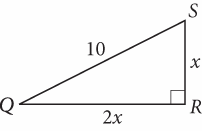In the figure above, what is the area of ΔQRS?

• A.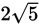• B. 10
• C.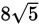• D. 20

Explanation:

D

Difficulty: Medium

Category: Additional Topics in Math / Geometry

Strategic Advice: Treat the variable lengths as you would number lengths; the same rules and theorems still apply.

Getting to the Answer: The area of a triangle is given by the formula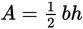. Before you can use the area formula, you'll need to use the Pythagorean theorem to find the lengths of the base and the height, 2x and x: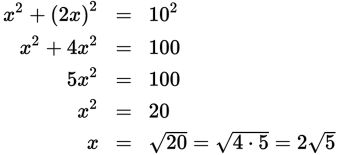The height (x) is, and the base is twice that, or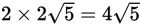. This makes the area of the triangle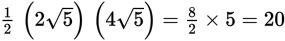, which matches (D).